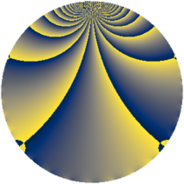# Properties

 Label 605.2.oLevel $605$ Weight $2$ Character orbit 605.o Rep. character $\chi_{605}(34,\cdot)$ Character field $\Q(\zeta_{22})$ Dimension $640$ Newform subspaces $1$ Sturm bound $132$ Trace bound $0$

# Related objects

## Defining parameters

 Level: $$N$$ $$=$$ $$605 = 5 \cdot 11^{2}$$ Weight: $$k$$ $$=$$ $$2$$ Character orbit: $$[\chi]$$ $$=$$ 605.o (of order $$22$$ and degree $$10$$) Character conductor: $$\operatorname{cond}(\chi)$$ $$=$$ $$605$$ Character field: $$\Q(\zeta_{22})$$ Newform subspaces: $$1$$ Sturm bound: $$132$$ Trace bound: $$0$$

## Dimensions

The following table gives the dimensions of various subspaces of $$M_{2}(605, [\chi])$$.

Total New Old
Modular forms 680 680 0
Cusp forms 640 640 0
Eisenstein series 40 40 0

## Trace form

 $$640q + 44q^{4} - 7q^{5} + 14q^{6} - 644q^{9} + O(q^{10})$$ $$640q + 44q^{4} - 7q^{5} + 14q^{6} - 644q^{9} - 23q^{10} + 4q^{11} - 30q^{14} + 18q^{15} - 100q^{16} - 6q^{19} - 3q^{20} + 32q^{21} + 128q^{24} - 15q^{25} - 26q^{26} - 10q^{29} + 28q^{30} - 18q^{31} - 34q^{34} - 29q^{35} - 66q^{36} + 44q^{39} - 50q^{40} - 34q^{41} - 28q^{44} - 43q^{45} - 14q^{46} + 102q^{49} + 29q^{50} - 148q^{51} + 90q^{54} - 102q^{55} - 106q^{56} - 34q^{59} + 58q^{60} - 42q^{61} + 24q^{64} + 22q^{65} + 52q^{66} + 20q^{69} - 75q^{70} + 54q^{71} - 34q^{74} - 4q^{75} - 2q^{76} + 50q^{79} - 160q^{80} + 560q^{81} - 4q^{84} - 57q^{85} + 6q^{86} - 128q^{89} + 39q^{90} - 80q^{91} - 88q^{94} + 65q^{95} + 8q^{96} - 266q^{99} + O(q^{100})$$

## Decomposition of $$S_{2}^{\mathrm{new}}(605, [\chi])$$ into newform subspaces

Label Dim. $$A$$ Field CM Traces $q$-expansion
$$a_2$$ $$a_3$$ $$a_5$$ $$a_7$$
605.2.o.a $$640$$ $$4.831$$ None $$0$$ $$0$$ $$-7$$ $$0$$